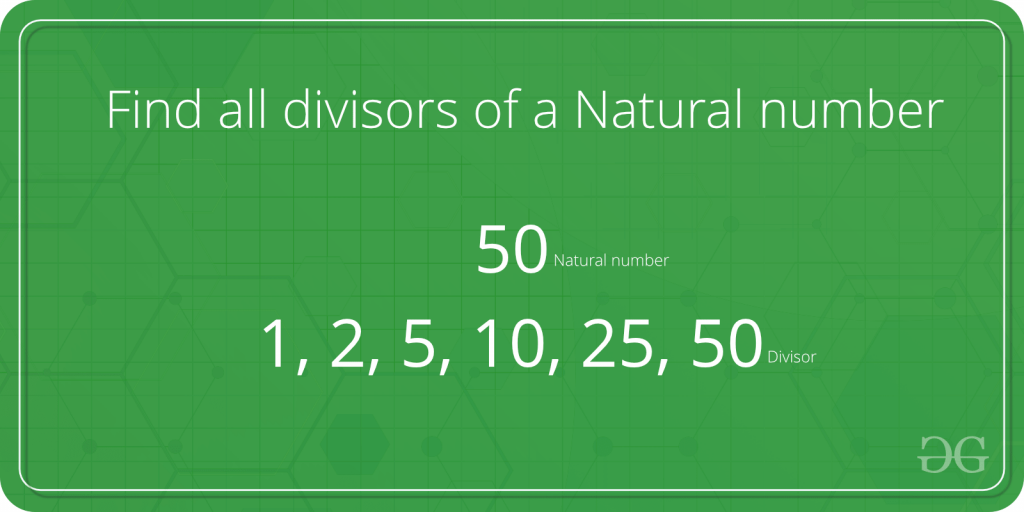Open in App
Not now

# C++ Program To Find All Factors of A Natural Number

• Last Updated : 17 Jan, 2023

Given a natural number n, print all distinct divisors of it.Examples:

``` Input : n = 10
Output: 1 2 5 10

Input:  n = 100
Output: 1 2 4 5 10 20 25 50 100

Input:  n = 125
Output: 1 5 25 125```

Note that this problem is different from finding all prime factors.

A Naive Solution would be to iterate all the numbers from 1 to n, checking if that number divides n and printing it. Below is a program for the same:

## C++

 `// C++ implementation of Naive  ` `// method to print all divisors ` `#include ` `using` `namespace` `std; ` ` `  `// Function to print the divisors ` `void` `printDivisors(``int` `n) ` `{ ` `    ``for` `(``int` `i = 1; i <= n; i++) ` `        ``if` `(n % i == 0) ` `            ``cout <<``" "` `<< i; ` `} ` ` `  `// Driver code ` `int` `main() ` `{ ` `    ``cout <<``"The divisors of 100 are: "``; ` `    ``printDivisors(100); ` `    ``return` `0; ` `} ` ` `  `// This code is contributed by shivanisinghss2110`

Output:

```The divisors of 100 are:
1 2 4 5 10 20 25 50 100```

Time Complexity : O(n)
Auxiliary Space : O(1)

Can we improve the above solution?
If we look carefully, all the divisors are present in pairs. For example if n = 100, then the various pairs of divisors are: (1,100), (2,50), (4,25), (5,20), (10,10)
Using this fact we could speed up our program significantly.
We, however, have to be careful if there are two equal divisors as in the case of (10, 10). In such case, we’d print only one of them.

Below is an implementation for the same:

## C++

 `// A Better (than Naive) Solution  ` `// to find all divisors ` `#include ` `#include ` `using` `namespace` `std; ` ` `  `// Function to print the divisors ` `void` `printDivisors(``int` `n) ` `{ ` `    ``// Note that this loop runs  ` `    ``// till square root ` `    ``for` `(``int` `i = 1; i <= ``sqrt``(n); i++) ` `    ``{ ` `        ``if` `(n % i == 0) ` `        ``{ ` `            ``// If divisors are equal,  ` `            ``// print only one ` `            ``if` `(n / i == i) ` `                ``cout <<``" "``<< i; ` ` `  `            ``// Otherwise print both ` `            ``else`  `                ``cout << ``" "``<< i << ``" "` `<< n / i; ` `        ``} ` `    ``} ` `} ` ` `  `// Driver code ` `int` `main() ` `{ ` `    ``cout <<``"The divisors of 100 are: "``; ` `    ``printDivisors(100); ` `    ``return` `0; ` `} ` ` `  `// This code is contributed by shivanisinghss2110`

Output:

```The divisors of 100 are:
1 100 2 50 4 25 5 20 10```

Time Complexity: O(sqrt(n))
Auxiliary Space : O(1)

However there is still a minor problem in the solution, can you guess?
Yes! the output is not in a sorted fashion which we had got using the brute-force technique. Please refer below for an O(sqrt(n)) time solution that prints divisors in sorted order.
Find all divisors of a natural number | Set 2

My Personal Notes arrow_drop_up
Related Articles